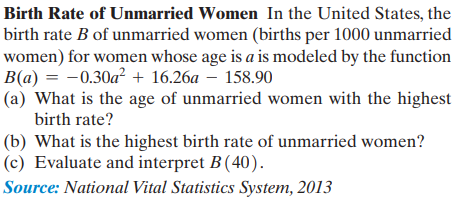### Still have math questions?

Algebra
QuestionBirth Rate of Unmarried Women

In the United States, the birth rate $$B$$ of unmarried women (births per $$1000$$ unmarried women) for women whose age is $$a$$ is modeled by the function

$$B ( a ) = - 0.30 a ^ { 2 } + 16.26 a - 158.90$$

(a) What is the age of unmarried women with the highest birth rate?

(b) What is the highest birth rate of unmarried women? (c) Evaluate and interpret $$B ( 40 )$$ .